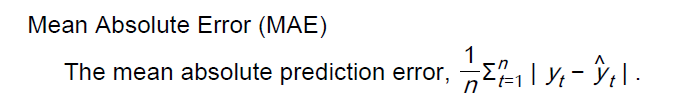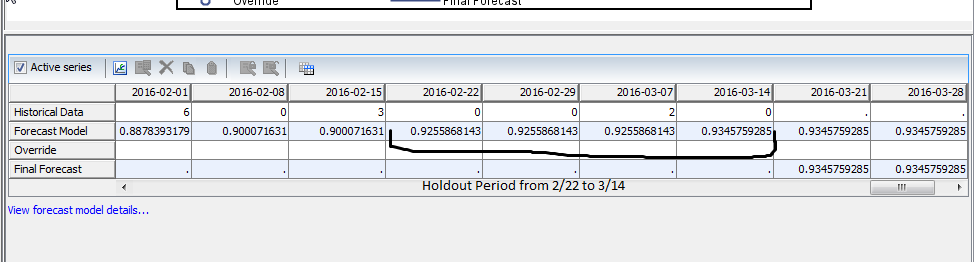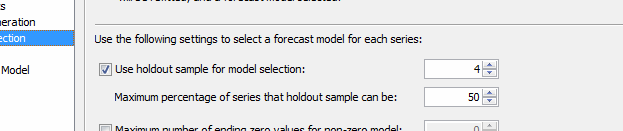## How does forecast server calculate error statistics for Intermittent forecast

Hi,

I'm fairly new to SAS.  I'm using Forecast Studio 12.1 for a Forecast Value Add analysis of another system's forecasts.  I'd like to know how SAS forecasts the MAE for intermittent demand forecasts.  For example, the Holdout MAE for one of my forecasts was 0, the forecast was 1 for all four cycles, and the holdout values were 0 2 0 2.  By my calculation this means the MAE is 4, but the 0 is a better representation of how well the forecast is doing.  What is the exact calculation for MAE that SAS is using?  I'd like to use the same calculation for my other system.  I think it is grouping together multiple weeks of demand into one "demand qty" for the forecast accuracy, but I'm not sure how it decides which weeks to group.  Is it based on a forecast "demand frequency" or on the actual next demand?

I've attached the SAS forecast studio guide definition of MAE.1 ACCEPTED SOLUTION

Accepted Solutions

## Re: How does forecast server calculate error statistics for Intermittent forecast

The forecasts showing in MAE2 image are not the forecasts used to compute holdout MAE. Holdout samples are used for model selection. To be specific, all candidate models are first built based on the data excluding the holdout samples.Forecasts are then be generated for the holdout samples to compute the holdout MAE. The model with the smallest MAE will be selected as the winning model. The winning model is then re-estimated using the entire dataset (assuming BACK option is disabled). Of course you can pick a different forecast error measurement for model selection. Hope this helps.

Alex

4 REPLIES 4udo_sas
SAS Employee

## Re: How does forecast server calculate error statistics for Intermittent forecast

Hello -

From SAS Forecast Server Procedures 14.1: User's Guide ("Forecasting Process Details"):

"Time-Indexed versus Demand-Indexed Holdout Samples

Holdout samples are typically specified based on the time index. But for intermittent demand model selection, demand-indexed-based holdouts are used for model selection. For example, consider “holdout the last six months data.” For a demand series, the demand indexed holdout refers to the “demands that have occurred in the last six months.” If there are four demands in the last six months, the demand indexed holdout is four for a time-indexed holdout of six. If there are no demands in the time-indexed holdout, the demand-indexed holdout is zero and in-sample analysis is used."

You will find additional details in the "Intermittent Models" chapter.

Thanks,

Udo

## Re: How does forecast server calculate error statistics for Intermittent forecast

Hi Udo.  Thank you for your answer.  Unfortunately, I'm still not getting the same numbers when I calculate it myself.  Here is an example.

Say I have a 4 week holdout for my non-intermittent demand.  So I'm looking at the demand in that period.

During these 4 weeks I have 1 nonzero demand (2 units) on the third week and my forecast is 0.9226 for the previous 3 weeks (see MAE2).  So I would say abs(3*.9226-2)/1=0.76 is the MAE.  However SAS says that the Holdout MAE is 0.006299.

I'm attaching screenshots of the MAE (MAE1), the forecast/history chart (MAE2), and the Holdout Size (MAE3).  Can you tell me where I'm going wrong here?  Thank you.

Edit: I tried to calculate the MAE for a few of the non-intermittent models as well and came up with different numbers.  So I'd appreciate any help you can give me understanding what I'm doing wrong. I also tried searching the forecast studio guide for the word intermittent, but there was no information about how error is calculated.  There is also no information on MAE beyond the formula: 1/n sum(abs(dmd-fcst))## Re: How does forecast server calculate error statistics for Intermittent forecast

The forecasts showing in MAE2 image are not the forecasts used to compute holdout MAE. Holdout samples are used for model selection. To be specific, all candidate models are first built based on the data excluding the holdout samples.Forecasts are then be generated for the holdout samples to compute the holdout MAE. The model with the smallest MAE will be selected as the winning model. The winning model is then re-estimated using the entire dataset (assuming BACK option is disabled). Of course you can pick a different forecast error measurement for model selection. Hope this helps.

Alex

## Re: How does forecast server calculate error statistics for Intermittent forecast

Yes.  That makes much more sense now.  Thank you!

Discussion stats
• 4 replies
• 1052 views
• 1 like
• 3 in conversation